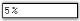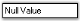# Value Settings in Windows Forms Percent TextBox

28 Apr 2021 / 2 minutes to read

The various values of the PercentTextBox control and their settings are given below.

``````this.percentTextBox1.PercentValue = 5;
this.percentTextBox1.DefaultValue = 0;
this.percentTextBox1.BindableValue = 0.05;
this.percentTextBox1.BindablePercentValue = 5;
this.percentTextBox1.DoubleValue = 0.05;``````
``````Me.percentTextBox1.PercentValue = 5
Me.percentTextBox1.DefaultValue = 0
Me.percentTextBox1.BindableValue = 0.05
Me.percentTextBox1.BindablePercentValue = 5
Me.percentTextBox1.DoubleValue = 0.05``````## Null value settings

There are various settings that can be applied to the PercentTextBox control, when the value of the control is set to ‘Null’. These settings are illustrated below.

``````this.percentTextBox1.NullString = "Null Value";
this.percentTextBox1.AllowNull = true;``````
``````Me.percentTextBox1.NullString = "Null Value"
Me.percentTextBox1.AllowNull = True``````## Min and max value settings

The minimum and maximum values of the IntegerTextBox can be set using the below given properties.

``````this.percentTextBox1.MaxValue = 6;
this.percentTextBox1.MinValue = -6;
this.percentTextBox1.EnforceMinMaxDuringValidating = true;``````
``````Me.percentTextBox1.MaxValue = 6
Me.percentTextBox1.MinValue = -6
Me.percentTextBox1.EnforceMinMaxDuringValidating = True``````

The methods associated with the above properties are given below.